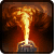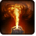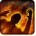# FlamethrowerConditions

Used by

## Related effects, buffs and debuffs

Please click on an effect below to view its details.

• [hidden] [does not expire]Effect #1

 Slot: Debuff Duration: passive Tick rate: 1s # occurrences: 1 Tags: tag.​abl.​utility.​is_aoe Target overrides: [target override] Aoe Sphere
• On Apply

Perform the following actions:

• Immobilize but allow rotation
• When effect is applied
• When effect ticks
• [target override]

Only when the following conditions are met:

• <
• If PRIMARY TARGET is alive
AND
• If target is an enemy
>

Perform the following actions:

• Add effect #2 to TARGET from TARGET, Combat Math Only
• Add effect #4 to TARGET from TARGET
• When channel ended

Perform the following actions:

• Add effect #3 to TARGET from TARGET
• Play appearance epp . npc . ability . skytrooper . firestorm_channel_exit, dependent on calling effect
• [5s]Burning
Periodic elemental damage.

 Slot: Debuff Duration: 5s Tick rate: 3s # occurrences: 1 Tags: tag.​abl.​debuff.​damage_over_time, tag.​abl.​debuff.​burn Conditions: Can only by called by other effects
• On Apply

Perform the following actions:

• Play appearance epp . bounty_hunter . firestorm . firestorm_burn_target, dependent on calling effect
• When effect ticks

Only when the following conditions are met:

• If difficulty is set to 16-man

Perform the following actions:

• Spell Damage
- Unknown (609) = (bool) false
- Damage Type = (int) 3
- Slot = (int) 1
- Spell Type = (int) 2
- Level Cap = (int) 0
- Amount Max = (float) 0
- Amount Min = (float) 0
- Amount Percent = (float) 0
- Threat Percent = (float) 0
- Standard Health Percent Max = (float) 0.015
- Standard Health Percent Min = (float) 0.015
- Amount Modifier Percent = (float) 0
- Coefficient = (float) 0
- Health Steal Percentage = (float) 0
• When effect ticks

Only when the following conditions are met:

• If difficulty is set to 8-man SM

Perform the following actions:

• Spell Damage
- Unknown (609) = (bool) false
- Damage Type = (int) 3
- Slot = (int) 1
- Spell Type = (int) 2
- Level Cap = (int) 0
- Amount Max = (float) 0
- Amount Min = (float) 0
- Amount Percent = (float) 0
- Threat Percent = (float) 0
- Standard Health Percent Max = (float) 0.015
- Standard Health Percent Min = (float) 0.015
- Amount Modifier Percent = (float) 0
- Coefficient = (float) 0
- Health Steal Percentage = (float) 0
• When effect ticks

Only when the following conditions are met:

• If difficulty is set to 8-man HM

Perform the following actions:

• Spell Damage
- Unknown (609) = (bool) false
- Damage Type = (int) 3
- Slot = (int) 1
- Spell Type = (int) 2
- Level Cap = (int) 0
- Amount Max = (float) 0
- Amount Min = (float) 0
- Amount Percent = (float) 0
- Threat Percent = (float) 0
- Standard Health Percent Max = (float) 0.015
- Standard Health Percent Min = (float) 0.015
- Amount Modifier Percent = (float) 0
- Coefficient = (float) 0
- Health Steal Percentage = (float) 0
• [hidden] [1.5s]Effect #3

 Slot: Debuff Duration: 1.5s Tick rate: does not tick # occurrences: 1 Conditions: Can only by called by other effects
• On Apply

Perform the following actions:

• Immobilize but allow rotation
• Global Cooldown
- Duration = (time) 1s
• [0s] [not displayed]Effect #4

 Slot: Debuff Duration: 0s Tick rate: does not tick # occurrences: 0 Conditions: Can only by called by other effects
• On Apply

Only when the following conditions are met:

• If difficulty is set to 16-man

Perform the following actions:

• Spell Damage
- Unknown (609) = (bool) false
- Damage Type = (int) 3
- Slot = (int) 1
- Spell Type = (int) 2
- Level Cap = (int) 0
- Amount Max = (float) 0
- Amount Min = (float) 0
- Amount Percent = (float) 0
- Threat Percent = (float) 0
- Standard Health Percent Max = (float) 0.03
- Standard Health Percent Min = (float) 0.03
- Amount Modifier Percent = (float) 0
- Coefficient = (float) 0
- Health Steal Percentage = (float) 0
• On Apply

Only when the following conditions are met:

• If difficulty is set to 8-man SM

Perform the following actions:

• Spell Damage
- Unknown (609) = (bool) false
- Damage Type = (int) 3
- Slot = (int) 1
- Spell Type = (int) 2
- Level Cap = (int) 0
- Amount Max = (float) 0
- Amount Min = (float) 0
- Amount Percent = (float) 0
- Threat Percent = (float) 0
- Standard Health Percent Max = (float) 0.035
- Standard Health Percent Min = (float) 0.035
- Amount Modifier Percent = (float) 0
- Coefficient = (float) 0
- Health Steal Percentage = (float) 0
• On Apply

Only when the following conditions are met:

• If difficulty is set to 8-man HM

Perform the following actions:

• Spell Damage
- Unknown (609) = (bool) false
- Damage Type = (int) 3
- Slot = (int) 1
- Spell Type = (int) 2
- Level Cap = (int) 0
- Amount Max = (float) 0
- Amount Min = (float) 0
- Amount Percent = (float) 0
- Threat Percent = (float) 0
- Standard Health Percent Max = (float) 0.03
- Standard Health Percent Min = (float) 0.03
- Amount Modifier Percent = (float) 0
- Coefficient = (float) 0
- Health Steal Percentage = (float) 0